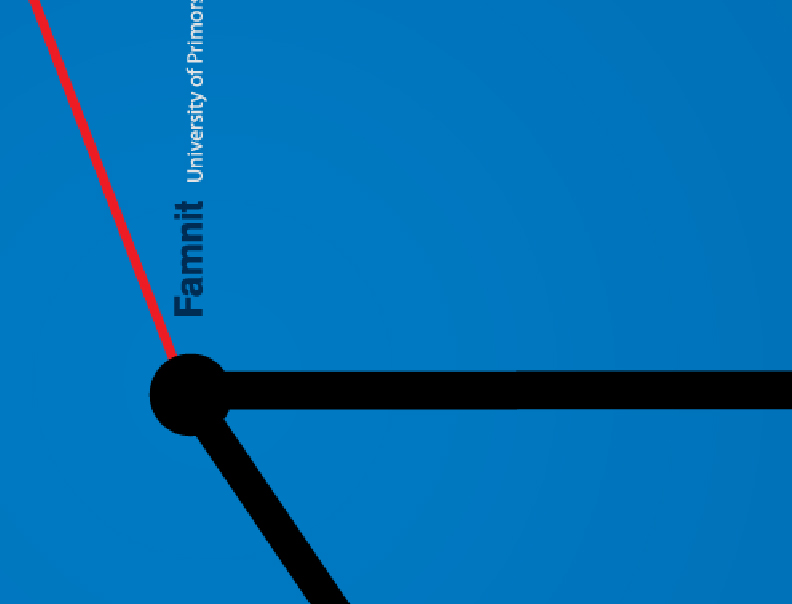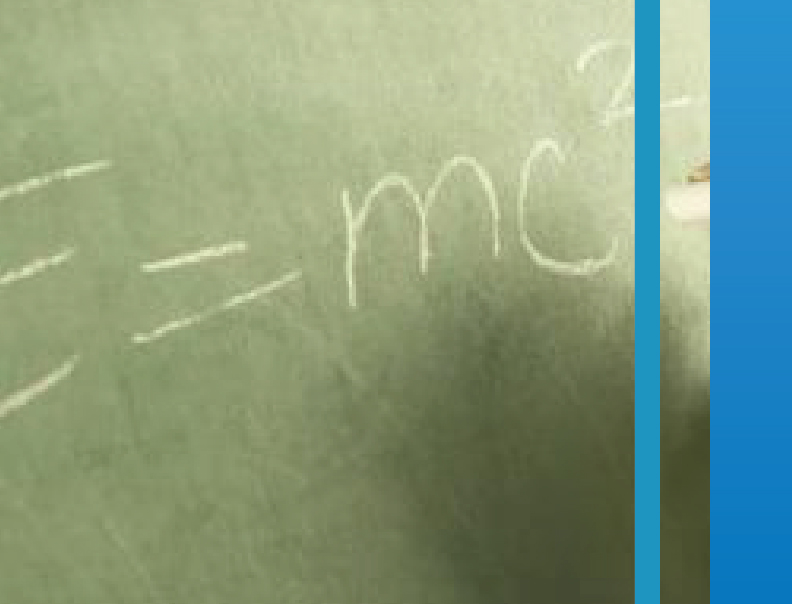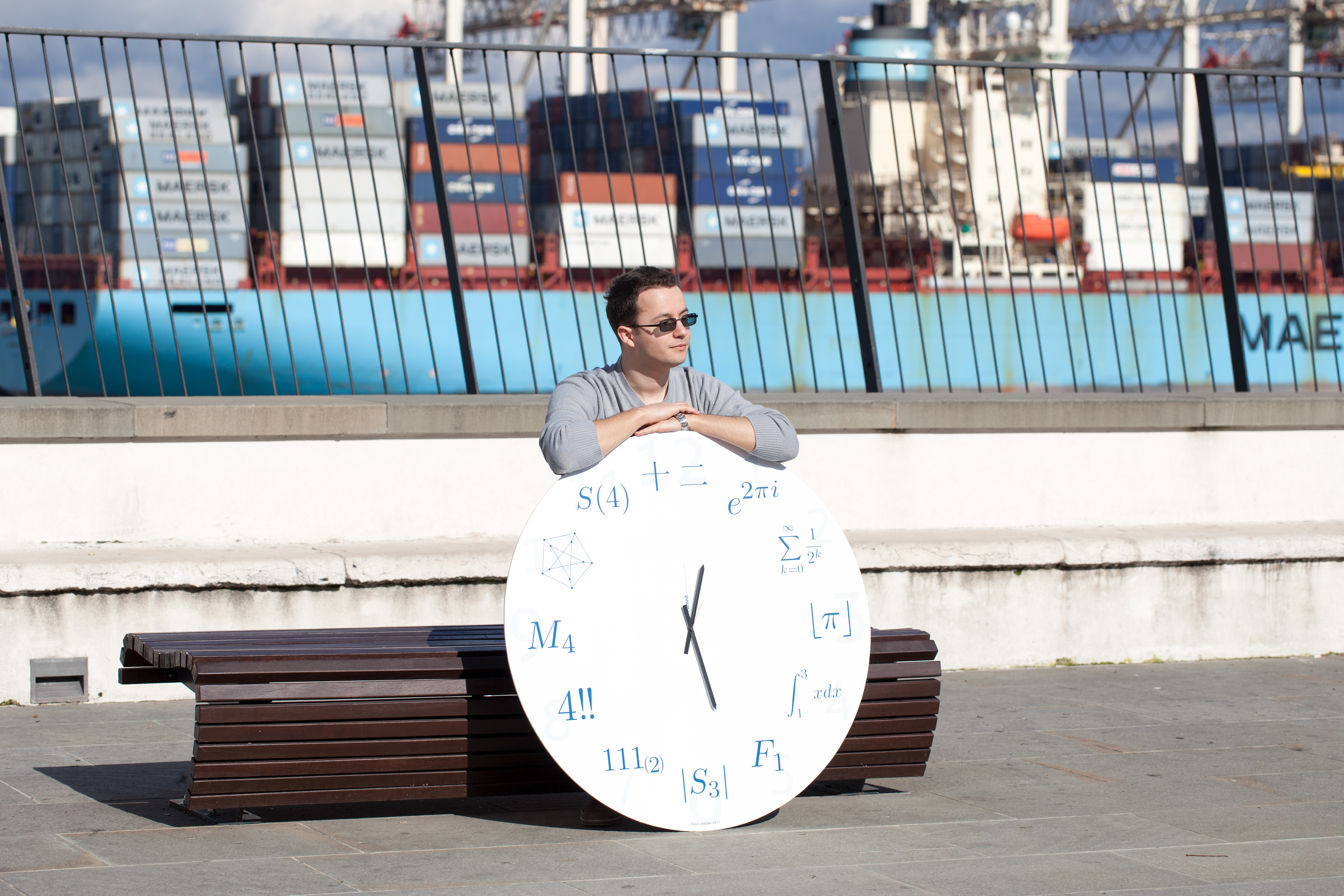Univerza na Primorskem Fakulteta za matematiko, naravoslovje in informacijske tehnologijeSI | EN# Raziskovalni matematični seminar - Arhiv

 2022 2021 2020 2019 2018 2017 2016 2015 2014 2013 2012 2011 2010
 1 2 3 4 5 6 7 8 9 10 11 12
Datum in ura / Date and time: 17.1.22
(10:00 -- 11:00)
Predavalnica / Location: ZOOM
Predavatelj / Lecturer: Mirza Krbezlija (UP FAMNIT, Slovenia)
Naslov / Title: Covering and domination at distance
Vsebina / Abstract:

Various theoretical and practical motivations have led to generalizations of many classical graph optimization problems to their distance-based variants. Informally, this means that the adjacency property used to define a feasible solution to the problem is replaced with a relaxed property based on distances in graphs. We consider distance-based variants of four classical covering and domination problems in graphs: the dominating set, edge dominating set, vertex cover, and edge cover problems. We study the relationships between the optimal solution values of the  corresponding problems and the algorithmic complexity of their computation under certain restrictions. More precisely, we study the Distance-k Dominating Set, Distance-k Edge Dominating Set, Distance-k Vertex Cover, and Distance-k Edge Cover problems. For each k ≥ 1 and for each of the four problems, we completely characterize the family of graphs H such that the problem is solvable in polynomial time in the class of H-free graphs (under the assumption that P \neq NP).

Joint work with Martin Milanič and Clément Dallard.

Our Math Research Seminar will only be broadcasted via Zoom.

We are looking forward to meeting at the video-conference.

Join the Zoom Meeting Here.

Everyone is welcome and encouraged to attend.

Datum in ura / Date and time: 10.1.22
(10:00 -- 11:00)
Predavalnica / Location: FAMNIT-MP1 & ZOOM
Predavatelj / Lecturer: Martin Milanič (UP FAMNIT and IAM, Slovenia)
Naslov / Title: Rise and fall of three conjectures on equistable graphs
Vsebina / Abstract:

In 1980, Payan introduced equistable graphs as graphs admitting positive weights on vertices such that a subset of vertices is a maximal stable set if and only if it is of total weight 1.

In 1993, McAvaney, Robertson, and DeTemple introduced general partition graphs as the intersection graphs of set systems over a finite ground set U such that every maximal stable set of the graph corresponds to a partition of U.

General partition graphs are exactly the graphs every edge of which is contained in a strong clique (that is, a clique intersecting all maximal stable sets).

In 1994, Mahadev, Peled, and Sun introduced strongly equistable graphs as graphs such that for every c ≤ 1 and every nonempty subset T of vertices that is not a maximal stable set, there exist positive vertex weights assigning weight 1 to every maximal stable set such that the total weight of T does not equal c. They proved that every strongly equistable graph is equistable, and conjectured that the converse holds as well.

In 2009, Orlin proved that every general partition graph is equistable, and conjectured that the converse holds as well. Orlin's conjecture, if true, would provide a combinatorial characterization of equistable graphs and imply the conjecture due to Mahadev, Peled, and Sun. An "intermediate" conjecture, posed by Miklavič and Milanič in 2011, states that every equistable graph has a strong clique.

The above conjectures have been verified for a number of graph classes.

In this talk we will present a construction, due to Milanič and Trotignon from 2017, of counterexamples to all three conjectures. The constructions are obtained within the class of complements of line graphs of triangle-free graphs and are based on connections with matchings and hamiltonicity. The same approach also leads to a separation between the classes of strongly equistable graphs and general partition graphs. We will also present several open questions.

Joint work with Nicolas Trotignon.

We are looking forward to meeting you at FAMNIT-MP1.

This Monday, January 10,  2022, from 10 am to 11 am.

Our Math Research Seminar will also be broadcasted via Zoom.

Join the Zoom Meeting Here.

See you there!

Everyone is welcome and encouraged to attend.